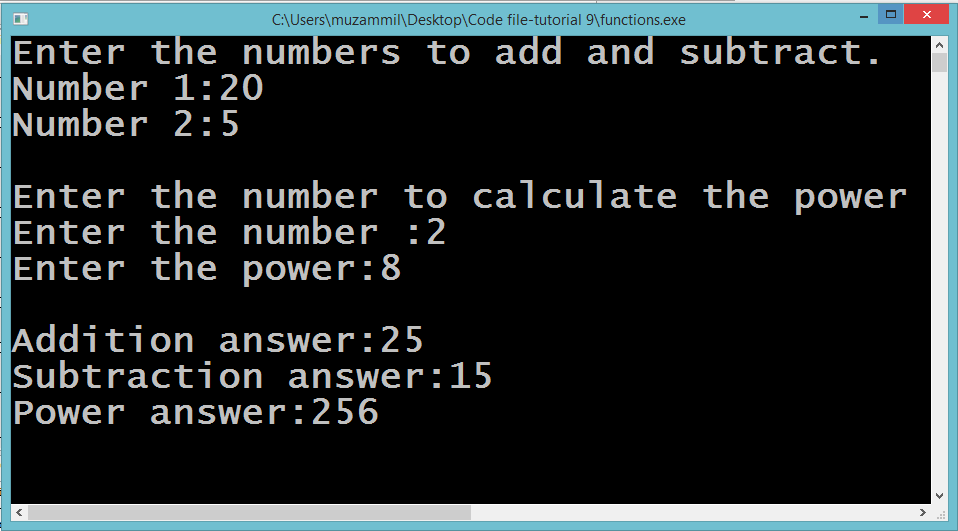# Introduction to Functions

## Introduction to Functions

In this part you will learn: 1. C syntax 2. Functions 3. Function Calling 4. Function definition 5. Function prototype 6. Showing output

#### What is a Function?

In C, a function is a group of statements that together performs a task. Every C program has at least one function called the ‘main()’ function. It is the heart of the program. Functions divide your code into separate small parts so that the code is easier to understand and easier to write. Each function performs a specific task based on its usage. The C language relies very much on function. The C standard library provides numerous built-in functions that your program can use. A function can be of different types. i.e. it can either have a return type, a name and some parameters or it can have no return type ‘ void’ and a name and parameters or it can only have a name but no return type or parameters. There is no restriction on the number of parameters a function can have. Calculating through Functions Basic Step: Open Dev C++ then File > new > source file and start writing the code below. These two are the most common and basic header files. The first one stdio.h is used to grant us most of the basic functions of C like Input and Output functions. The second one conio.h provides us functions like getch() which is used to pause our screen until we press a button.
`#include<stdio.h>#include<conio.h> int AddNumbers(int m1,int n1);int SubNumbers(int m1,int n1);int calculatePower(int n,int p);`
When using functions we have to first declare the function prototypes. This declaration is done before we start writing the main function. In the declaration we have to mention the return type of our functions. After that the name of the function whatever we like. But then it should be same everywhere. Just for making the function names significant we write the first letter of the name capital and any other word that comes in the function name. Then we mention our parameters that will be passed to our function. We have to mention the type of the parameters as well as assign them a name so that we can use these names in the body of the function.
`int main(){	int m,n;	printf("Enter the numbers to add and subtract.\n");	printf("Number 1:");	scanf(" %d",&m); 	printf("Number 2:");	scanf(" %d",&n); 	int ans1=AddNumbers(m,n);	int ans2=SubNumbers(m,n);`
Now we enter our main() function. Here we first declare some integers and take input in them from the user. After taking the input comes our first function call. When we write the name of our function in the main function that is called function calling. When we call a function we send in the variables as parameters that we need to send in order to perform our required task. When we call a function the control of our program goes inside the function. The body of the function is executed first and then the program returns to the main and the rest of the program is then executed. Now when we called the function we sent the numbers in the function as parameter the afterward the function returns the answer. Same is the case with the other function call.
`int num,pow;	printf("\nEnter the number to calculate the power");	printf("\nEnter the number :");	scanf(" %d",&num); 	printf("Enter the power:");	scanf(" %d",&pow); 	int ans3=calculatePower(num,pow);`
In this piece of the code we declared two more variables. These variables are for calculating the power of user entered number. We took input the number and the power from the user. Then we called the power function and sent the number and the power as the parameter. This function will calculate the power and return the ans which will be stored in the ans3 varibale.
`	printf("\nAddition answer:%d",ans1);	printf("\nSubtraction answer:%d",ans2);	printf("\nPower answer:%d",ans3);  	getch(); }`
At the end of thr program we just printed our three answers on the screen and called the getch() function which pauses the console screen.
`int AddNumbers(int m1,int n1){	int answer= m1+n1;	return answer;} int SubNumbers(int m1,int n1){	int answer= m1-n1;	return answer;} int calculatePower(int n,int p){	int product=1,i;	for(i=0;i<p;i++)	{		product=product*n; 	} 	return product;}`
Now these are our function definitions. These our written outside the main() function. Function definition contains all the code that needs to be executed when that specific function is called. The AddNumbers() function was called in main and we sent two variables to it. All this function did was added these numbers and stored them in a new variable and then it returned integer value of the result. The SubNumbers() function was called in main and we sent two variables to it. All this function did was subtracted these numbers and stored them in a new variable and then it returned integer value of the result. Same is the case with calculatePower() function. It also calculate the result and returns it. Execute > compile then Execute > run Output